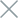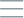• Home
• Tags
• Series
•# Cloud Search Algorithm in PHP: Explained with Example

The Cloud Search Algorithm is an advanced technique in PHP programming, used to search for potential solutions within a search space by employing the concept of "cloud" of solutions. It draws inspiration from how clouds in nature move across different areas to find sources of sustenance.

## How the Cloud Search Algorithm Works

The Cloud Search Algorithm begins by generating a large number of random solutions within the search space. These solutions are referred to as "solution particles." The algorithm then employs transformations and evaluations to move these solution particles through the search space.

• Integrates Exploration and Optimization: This algorithm combines the ability to explore a wide search space with the capability to optimize solutions.

• Parameter Consideration Required: The Cloud Search Algorithm demands careful consideration of setting parameters for generating solution particles and their movement through the search space.

## Example and Explanation

Consider an example of finding the minimum value of a mathematical function using the Cloud Search Algorithm in PHP.

``````function cloudSearch(\$numParticles, \$maxIterations) {
// Initialize particles randomly
\$particles = array();
for (\$i = 0; \$i < \$numParticles; \$i++) {
\$particles[\$i] = rand(-100, 100);
}

// Main optimization loop
for (\$iteration = 0; \$iteration < \$maxIterations; \$iteration++) {
foreach (\$particles as \$index => \$particle) {
// Apply transformations and evaluate fitness
// Update particle's position
}
}

// Return the best solution found
return min(\$particles);
}

\$numParticles = 50;
\$maxIterations = 100;

\$minimumValue = cloudSearch(\$numParticles, \$maxIterations);
echo "Minimum value found: \$minimumValue";
``````

In this example, we use the Cloud Search Algorithm to find the minimum value of a mathematical function by optimizing solution particles. Each solution particle is represented by a random value, and the algorithm uses transformations and evaluations to shift these solution particles through the search space. The result is the minimum value found through the optimization process.

While this example demonstrates how the Cloud Search Algorithm can be used to optimize a mathematical function, it can also be applied to other optimization problems in PHP.